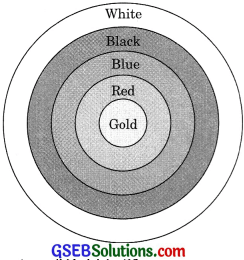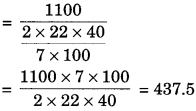# GSEB Solutions Class 10 Maths Chapter 12 Areas Related to Circles Ex 12.1

Gujarat Board GSEB Solutions Class 10 Maths Chapter 12 Areas Related to Circles Ex 12.1Textbook Questions and Answers.

## Gujarat Board Textbook Solutions Class 10 Maths Chapter 12 Areas Related to Circles Ex 12.1

Question 1.
The radii of two circles are 19 cm and 9 cm respectively. Find the radius of the circle which has a circumference equal to the sum of the circumferences of the two circles.
Solution:
Let radii of two circles be r1 and r2, and R be the radius of third circle whose circumference is equal to the sum of circumference of two circle
r1 = 19 cm
r2 = 9 cm
C1 + C2 = C
2πr2 + 2πr2 = 2πR
⇒ 2π(r1 + r2) = 2πR
⇒ r1 + r1 = R
⇒ 19 + 9 = R
⇒ R = 28 cm

Question 2.
The radii of two circles are 8 cm and 6 cm respectively. Find the radius of the circle having an area equal to the sum of the areas of the two circles.
Solution:
Let r1 and r2 be the area of two circles and radius of the third circle be R.
r1 = 8 cm, r2 = 6 cm
A1 + A2 = A
⇒ πr21 + πr22 = πR2
⇒ π(r21 + r22) = πR2
⇒ r21 + r22 = R2
⇒ 82 + 62 = R2
⇒ R2 = 64 + 36 = 100
⇒ R = 10 cmQuestion 3.
The figure depicts an archery target marked with its five scoring regions from the center outwards as Gold, Red, Blue, Black, and White. The diameter of the region representing the Gold score 21 cm and each of the other bands is 10.5 cm wide. Find the area of each of the five scoring regions.Solution:
Diameter of Gold is 21 cm
R1 = $$\frac {21}{2}$$ cm = 10.5 cm
Area of gold scoring region = πR21
= $$\frac {22}{7}$$ x (10.5)2
= $$\frac {22}{7}$$ x 10.5 x 10.5
= 346.5 cm2
R2 = R1 + 10.5 10.5 + 10.5 = 21 cm
Area of red scoring region = π [R22 – R21]
= $$\frac {22}{7}$$ [(21)2 – (10.5)2]
= $$\frac {22}{7}$$ x (21 + 10.5) (21 – 10.5)
= 22 x 4.5 x 10.5 = 1039.5 cm2
R3 = R2 + 10.5 = 31.5 cm
Area of blue scoring region
= π[R23 – R22]
= $$\frac {22}{7}$$ [(31.5)2 – (21)2]
= $$\frac {22}{7}$$ x (31.5 + 21) (31.5 – 21)
= $$\frac {22}{7}$$ x 7.5 x 10.5
R4 = R3 + 10.5 = 42
Area of black scoring region
= π[R24 – R23]
= $$\frac {22}{7}$$ (422 – 31.52)
= $$\frac {22}{7}$$ (42 + 31.5) (42 – 31.5)
= $$\frac {22}{7}$$ x 73.5 x 10.5
= 22 x 10.5 x 10.5
= 2425.5 cm2
Area of white scoring region
= π[R25 – R24]
= $$\frac {22}{7}$$ [(R4 + 10.5)2 – R24]
= $$\frac {22}{7}$$ (52.52 – 422]
= $$\frac {22}{7}$$ (52.5 + 42) (52.5 – 42)
= $$\frac {22}{7}$$ x 94.5 x 10.5
= 22 x 13.5 x 10.5
= 3118.25 cm2Question 4.
The wheels of a car are of a diameter of 80 cm each. How many complete revolutions does each wheel make in 10 minutes? When the car is traveling at a speed of 66 km per hour?
Solution:
Diameter of wheel = 80 cm
Radius of wheel r = $$\frac {80}{2}$$ = 40 cm
Distance covered by wheel in one revolution = 2πr
= 2 x $$\frac {22}{7}$$ x 40 cm
= $$\frac{2 \times 22 \times 40}{7 \times 100}$$ m
Distance covered by wheel in one minute
= $$\frac{66 \mathrm{km}}{60 \text { minute }}$$ = $$\frac{66 × 1000}{60}$$m
= $$\frac{66000}{60}$$m
= 1100 m
Number of complete revolutions in 1 minuteNumber of complete revolution in 10 minutes
= 437.5 x 10 = 4375Question 5.
Tick the correct answer in the following and justify your choice. If the perimeter and area of a circle are numerically equal, then the radius of the circle is –
(a) 2 units
(b) π units
(c) 4 units
(d) 7 units
Solution:
Let the radius of the circle be r units. Then according to question
Area of circle = Perimeter of the circle
πr2 = 2πr
r = 2 units
Hence, the correct answer is (a) 2 units.